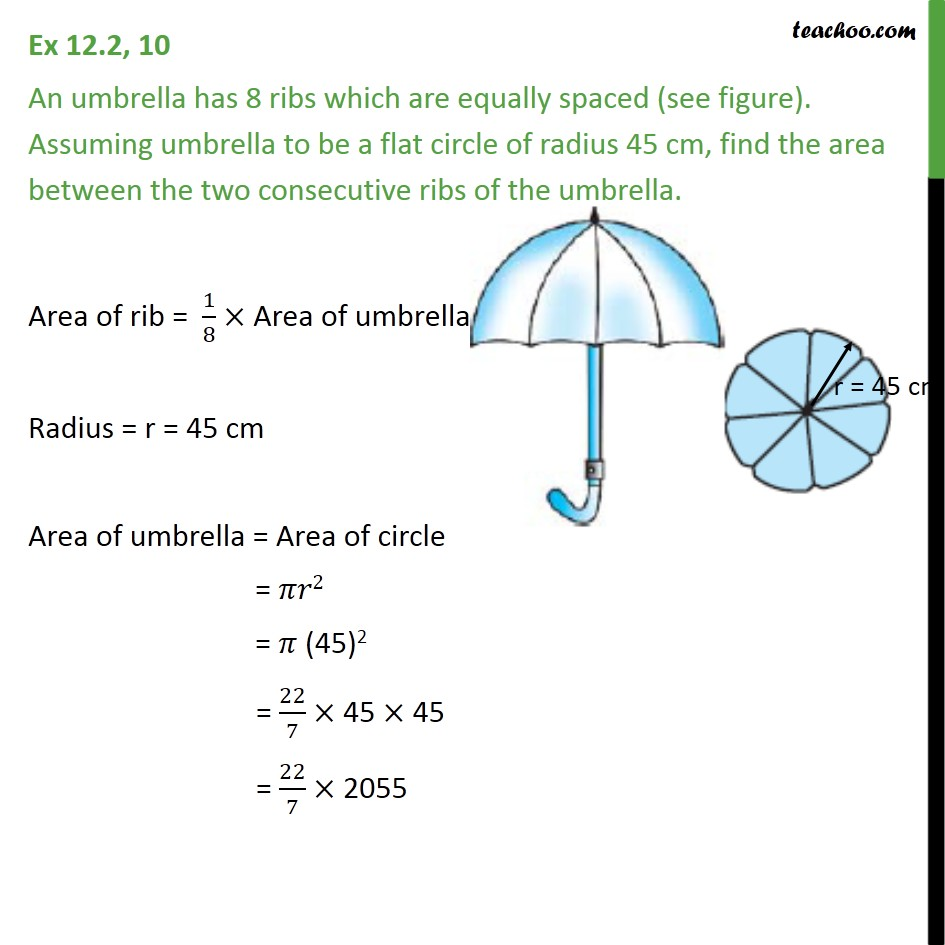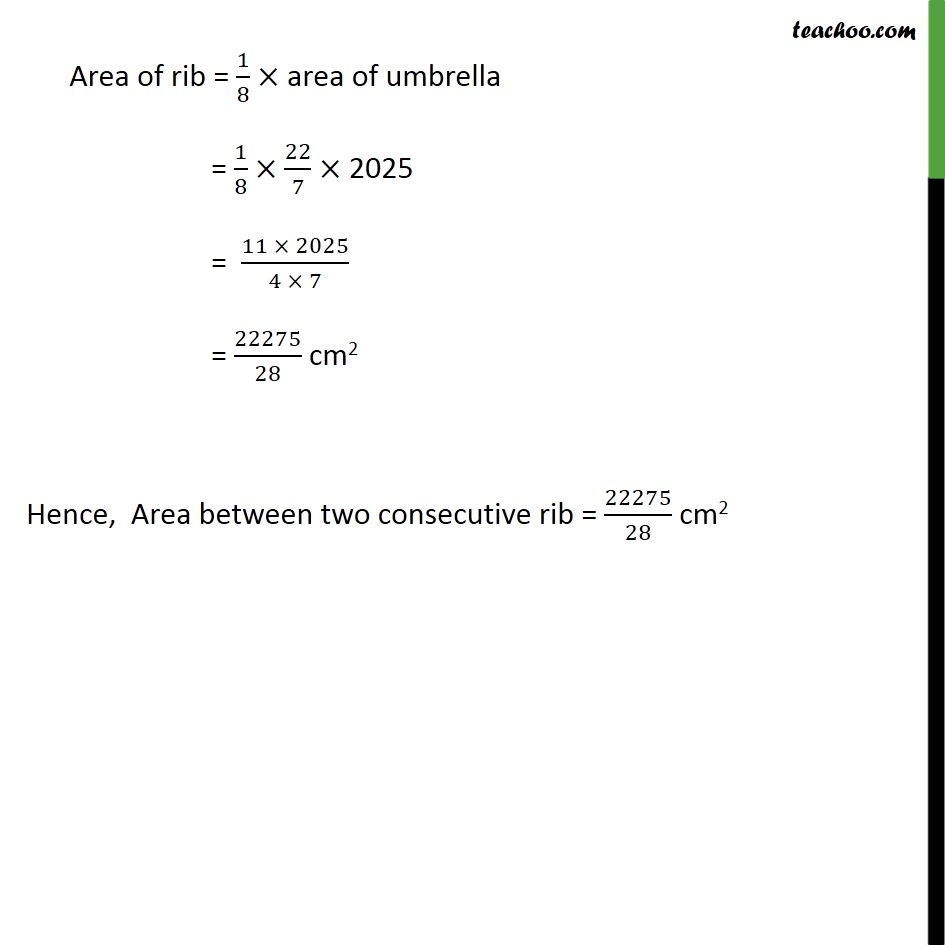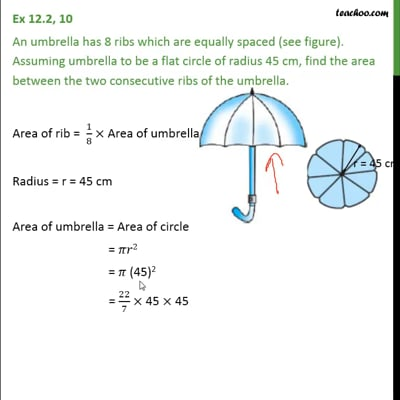Ex 12.2

Chapter 12 Class 10 Areas related to Circles
Serial order wiseThis video is only available for Teachoo black users

Introducing your new favourite teacher - Teachoo Black, at only ₹83 per month

### Transcript

Ex 12.2, 10 An umbrella has 8 ribs which are equally spaced (see figure). Assuming umbrella to be a flat circle of radius 45 cm, find the area between the two consecutive ribs of the umbrella. Area of rib = 1/8 Area of umbrella Radius = r = 45 cm Area of umbrella = Area of circle = 2 = (45)2 = 22/7 "45" 45 = 22/7 2055 Area of rib = 1/8 area of umbrella = 1/8 22/7 2025 = (11 2025)/(4 7) = 22275/28 cm2 Hence, Area between two consecutive rib = 22275/28 cm2